# Section 9.2. One-Dimensional Arrays

[Page 406 (continued)]

### 9.2. One-Dimensional Arrays

An array is a data structure. A data structure is an organized collection of data. In an array, data are arranged in a linear or sequential structure, with one element following another. When referencing elements in an array, we refer to the positions of the particular elements within the array. For example, if the array is named arr, then the elements are named arr, arr, arr, ... arr[n-1], where n gives the number of elements in the array. This naming also reflects the fact that the array's data are contained in storage locations that are next to each other. In Java, as in C, C++, and some other programming languages, the first element of an array has index 0. (This is the same convention we used for Strings.)

[Page 407]

Zero indexing

Figure 9.1 shows an array named arr that contains 15 int elements. The syntax for referring to elements of an array is

##### Figure 9.1. An array of 15 integers named arr.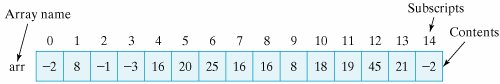arrayname [ subscript ]

where arrayname is the name of the arrayany valid identifier will doand subscript is the position of the element within the array. As Figure 9.1 shows, the first element in the array has subscript 0, the second has subscript 1, and so on.

A subscript is an integer quantity contained in square brackets that is used to identify an array element. An subscript must be either an integer value or an integer expression. Using Figure 9.1 to illustrate an example, suppose that j and k are integer variables equaling 5 and 7, respectively. Each of the following would then be a valid reference to elements of the array arr:

`arr     // Refers to 16 arr[j]     // Is arr which refers to 20 arr[j + k] // Is arr[5+7] which is arr which refers to 45 arr[k % j] // Is arr[7%5] which is arr which refers to -1 `

Subscript expressions

These examples show that when an expression, such as j + k, is used as a subscript, it is evaluated (to 12 in this case) before the reference is made.

It is a syntax error to use a noninteger type as an array subscript. Both of the following expressions would be invalid:

`arr[5.0]  // 5.0 is a float and can't be an array subscript arr["5"]  // "5" is a string not an integer `

For a given array, a valid array subscript must be in the range 0 ... N-1, where N is the number of elements in the array, and if not it, is considered out-of-bounds. An out-of-bounds subscript creates a runtime errorthat is, an error that occurs when the program is runningrather than a syntax error, which can be detected when the program is compiled. For the array arr, all of the following expressions contain out-of-bounds subscripts:

[Page 408]

`arr[-1]     // Arrays cannot have negative subscripts arr['5']    // Char '5' promoted to its Unicode value, 53 arr     // The last element of arr has subscript 14 arr[j*k]    // Since j*k equals 35 `

Each of these references would lead to an IndexOutOfBoundsException. (Exceptions are covered in detail in Chapter 10.)

Java Language Rule: Array Subscripts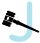Array subscripts must be integer values in the range 0. . . (N-1), where N is the number of elements in the array.

Debugging Tip: Array Subscripts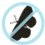In developing array algorithms, it is important to design test data that show that array subscripts do not cause runtime errors.

#### 9.2.1. Declaring and Creating Arrays

For the most part, arrays in Java are treated as objects. Like objects, they are instantiated with the new operator and they have instance variables (for example, length). Like variables for objects, array variables are considered reference variables. When arrays are used as parameters, a reference to the array is passed rather than a copy of the entire array. The primary difference between arrays and full-fledged objects is that arrays are not defined in terms of an Array class. Thus, arrays do not fit into Java's Object hierarchy. They do not inherit any properties from Object, and they cannot be subclassed.

Are arrays objects?

You can think of an array as a container that contains a number of variables. As we have seen, the variables contained in an array object are not referenced by name but by their relative positions in the array. The variables are called components. If an array object has N components, then we say that the array length is N. Each of the components of the array has the same type, which is called the array's component type. An empty array is one that contains zero variables.

Components and elements

A one-dimensional array has components that are called the array's elements. Their type is the array's element type. An array's elements may be of any type, including primitive and reference types. This means you can have arrays of int, char, boolean, String, Object, Image, TextField, TwoPlayerGame, and so on.

When declaring a one-dimensional array, you have to indicate both the array's element type and its length. Just as in declaring and creating other kinds of objects, creating an array object requires that we create both a name for the array and then the array itself. The following statements create the array shown in Figure 9.1:

`int arr[];          // Declare a name for the array arr = new int;  // Create the array itself `

[Page 409]

These two steps can be combined into a single statement, as follows:

`int arr[] = new int; `

In this example, the array's element type is int and its length is 15, which is fixed and cannot be changed. This means that the array contains 15 variables of type int, which will be referred to as arr, arr, . . . arr.

#### 9.2.2. Array Allocation

Creating the array in Figure 9.1 means allocating 15 storage locations that can store integers. One difference between declaring an array and declaring some other kind of object is that square brackets ([]) are used to declare an array type. The brackets can be attached either to the array's name or to its type, as in the following examples:

`int arr[];    // The brackets may follow the array's name int[] arr;    // The brackets may follow the array's type `

Allocating memory

The next example creates an array of five Strings and then uses a for loop to assign the strings "hello1", "hello2", "hello3", "hello4", and "hello5" to the five array locations:

`String strarr[];                             // Declare a name for the array strarr = new String;                      // Create the array itself                                              // Assign strings to the array for (int k = 0; k < strarr.length; k++)      // For each element   strarr[k] = new String("hello" + (k + 1)); // Assign a string `

The expression k < strarr.length specifies the loop bound. Every array has a length instance variable, which refers to the number of elements contained in the array. As we mentioned, arrays, like Strings, are zero indexed, so the last element of the array is always given by its length-1. However, length is an instance variable for arrays, whereas length() is an instance method for Strings. Therefore, it would be a syntax error in this example to refer to strarr.length().

length vs. length()

Debugging Tip: Array LengthA common syntax error involves forgetting that for arrays length is an instance variable, not an instance method, as it is for Strings.

In the example, we first use the new operator to create strarr, an array of type String of length 5. We then use a String constructor to create the five Strings that are stored in the array. It is important to realize that creating an array to store five Objects (as opposed to five primitive data elements) does not also create the Objects that will be stored in the array.

When an array of objects is created, the array's elements are references to those objects (Fig. 9.2). Their initial values, like all reference variables, are null. So to create and initialize the array strarr, we need to create six objectsthe array itself, which will contain five Strings, and then the five Strings that will be stored in strarr.

##### Figure 9.2. Creating an array of five Strings involves six objects, because the array itself is a separate object. In (a), the array variable is declared. In (b), the array is instantiated, creating an array of five null references. In (c), the five Strings are created and assigned to the array. (This item is displayed on page 410 in the print version)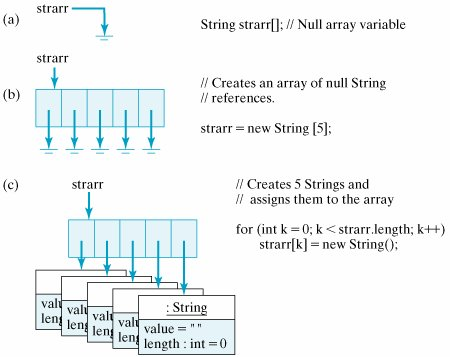Arrays of objects

[Page 410]

One more example will underscore this point. The following statements create four new Objects, an array to store three Students plus the three Students themselves:

`Student school[] = new Student;    // A 3 Student array school = new Student("Socrates");  // The first Student school = new Student("Plato");     // The second Student school = new Student("Aristotle"); // The third Student `

The first statement creates an array named school to store three Students, and the next three statements create the individual Students and assign them to the array (Fig. 9.3). Thus, creating the array and initializing its elements require four new statements. The following sequence of statements would lead to a null pointer exception because the array's elements have not been instantiated:

`Student students[] = new Student;        // A 3 Student array System.out.println(students.getName()); `

##### Figure 9.3. An array of Students. (This item is displayed on page 411 in the print version)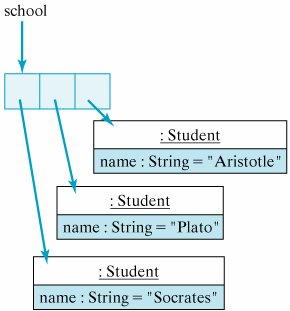In this case, students is a null reference, thus causing the exception.

Debugging Tip: Array InstantiationCreating a new array does not also create the objects stored in the array. They must be instantiated separately. It is a semantic error to refer to an uninstantiated (null) array element.

[Page 411]

Now that we have assigned the three Students to the array, we can refer to them by means of subscripted references. A reference to the Student named "Socrates" is now school, and a reference to the Student named "Plato" is school. In other words, to refer to the three individual students, we must refer to their locations within school. Of course, we can also use variables, such as loop counters, to refer to a Student's location within school. The following for loop invokes each Student's getState() method to print out its current state:

`for (int k = 0; k < school.length; k++)     System.out.println(school[k].getState()); `

What if the three Students already existed before the array was created? In that case, we could just assign their references to the array elements, as in the following example:

`Student student1 = new Student("Socrates"); Student student2 = new Student("Plato"); Student student3 = new Student("Aristotle"); Student school = new Student;             // A 3 Student array school = student1; school = student2; school = student3; `

In this case, each of the three Student objects can be referenced by two different referencesits variable identifier (such as student1) and its array location (such as school). For arrays of objects, Java stores just the reference to the object in the array rather than the entire object. This conserves memory, since references require only 4 bytes each, whereas each object may require hundreds of bytes (Fig. 9.4).

##### Figure 9.4. Arrays of objects store references to the objects, not the objects themselves. (This item is displayed on page 412 in the print version)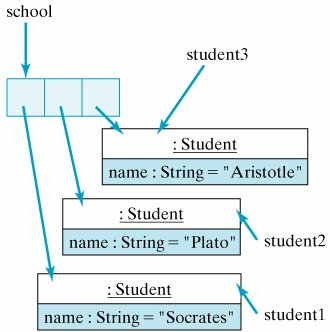When an array of N elements is created, the compiler allocates storage for N variables of the element's type. In the case of arr that we discussed earlier, the compiler would allocate storage for 15 ints60 contiguous bytes of storage, because each int requires 4 bytes (32 bits) of storage. If we declare an array of 20 doubles,

`double arr[] = new double; `

[Page 412]

the compiler will allocate 160 bytes of storage20 variables of 8 bytes (64 bits) each. In the case of the Student examples and the String examples, because these are objects (not primitive types), the compiler will allocate space for N addresses, where N is the length of the array and each address requires 4 bytes.

How much memory?

##### Self-Study Exercise

 Exercise 9.1 How much space (in bytes) would be allocated for each of the following? int a[] = new int;double b[] = new double;char c[] = new char;String s[] = new String;Student p[] = new Student;

#### 9.2.3. Initializing Arrays

Array elements are automatically initialized to default values that depend on the element type. Boolean elements are initialized to false, and integer and real types are initialized to 0. Reference typesthat is, arrays of objectsare initialized to null.

Default initialization

Arrays can also be assigned initial values when they are created, although this is feasible only for relatively small arrays. An array initializer is written as a list of expressions separated by commas and enclosed by braces. For example, we can declare and initialize the array shown in Figure 9.1 with the following statement:

`int arr[] = {-2,8,-1,-3,16,20,25,16,16,8,18,19,45,21,-2}; `

Array initializer

Similarly, to create and initialize an array of Strings, we can use the following statement:

`String strings[] = {"hello", "world", "goodbye", "love"}; `

[Page 413]

This example creates and stores four Strings in the array. Subsequently, to refer to "hello", we would use the reference strings, and to refer to "love", we would use the reference strings. Note in these examples that when an array declaration contains an initializer, it is not necessary to use new and it is not necessary to specify the number of elements in the array. The number of elements is determined from the number of values in the initializer list.

#### 9.2.4. Assigning and Using Array Values

Array elements can be used in the same way as other variables. The only difference, of course, is that references to elements are subscripted. For example, the following assignment statements assign values to the elements of two arrays, named arr and strings:

`arr = 5; arr = 10; arr = 3; strings = "who"; strings = "what"; strings = strings = "where"; `

Array assignment

The following loop assigns the first 15 squares1, 4, 9 . . .to the array arr:

`for (int k = 0; k < arr.length; k++)     arr[k] = (k+1) * (k+1); `

The following loop prints the values of the array arr:

`for (int k = 0; k < arr.length; k++)     System.out.println(arr[k]); `

##### Self-Study Exercises

 Exercise 9.2 Declare an array named farr that contains ten floats initialized to the values 1.0, 2.0, . . . , 10.0. Exercise 9.3 Write an expression that prints the first element of farr. Exercise 9.4 Write an assignment statement that assigns 100.0 to the last element in farr. Exercise 9.5 Write a loop to print all of the elements of farr.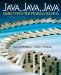Java, Java, Java, Object-Oriented Problem Solving (3rd Edition)
ISBN: 0131474340
EAN: 2147483647
Year: 2005
Pages: 275

Similar book on Amazon